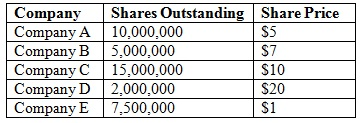# Price-Weighted Index

Posted in Finance, Accounting and Economics Terms, Total Reads: 874

## Definition: Price-Weighted Index

Price-weighted index is an index in which each stock affects the index in relation to its price/share. The index value is calculated by summing the prices of each stock in the index and dividing them by the total number of stocks outstanding. Stocks with a greater price will be given greater weight and, therefore, will have a more influence on the performance of the index.

For example, let's assume that the following companies are in the ABC price-weighted index:A price-weighted index is basically the sum of the members' stock prices divided by the number of members. Thus, here our example, the ABC index is: \$5 + \$7 + \$10 + \$20 + \$1 = \$43 / 5 = 8.6.

Browse the definition and meaning of more terms similar to Price-Weighted Index. The Management Dictionary covers over 7000 business concepts from 6 categories.

Search & Explore : Management Dictionary

Similar Definitions from same Category: